MATLAB Function Referenceasin

Inverse sine, result in radians

Syntax

• ```Y = asin(X)
```

Description

```Y = asin(X) ``` returns the inverse sine (arcsine) for each element of `X`. For real elements of `X` in the domain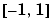, `asin(X)` is in the range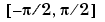. For real elements of `x` outside the range, `asin(X)` is complex.

The `asin` function operates element-wise on arrays. The function's domains and ranges include complex values. All angles are in radians.

Examples

Graph the inverse sine function over the domain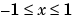.

• ```x = -1:.01:1;
plot(x,asin(x)), grid on
```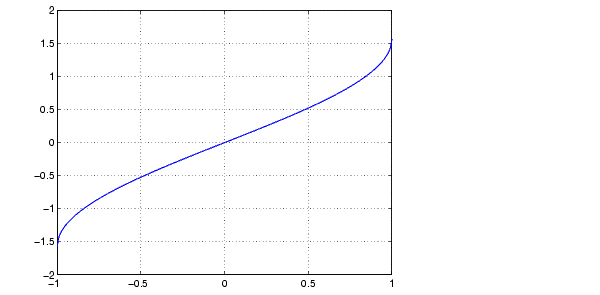Definition

The inverse sine can be defined as

•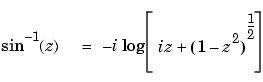Algorithm

`asin` uses FDLIBM, which was developed at SunSoft, a Sun Microsystems, Inc. business, by Kwok C. Ng, and others. For information about FDLIBM, see http://www.netlib.org.

See Also

`sin`, `asind`, `asinh`

© 1994-2005 The MathWorks, Inc.